# Python Program to Count Vowels, Lines, and Characters in a Text File

This article is created to cover many programs in Python related to counting characters such as vowels, consonants, newlines, blank spaces, etc. in a given or entered text file by the user at run-time. Here is a list of programs covered in this article:

• Count vowels in a text file
• Count consonants in a text file
• Count new lines in a text file
• Count blank spaces in a text file
• Count the total or all characters in a text file

Before moving on to the programs, let's do some important things to implement and execute the program based on the text file.

### Things to Do Before the Program

Because the program given below is used to count characters in a text file, therefore, first we've got to create a text file called "codescracker.txt" with some contents like:

```Hello Python
This is a text File
The name of this file is codescracker.txt```

Save this file in the current directory. The current directory is the directory where the Python code to count characters in this file is saved. Here is a snapshot of the folder where the file "codescracker.txt" is saved: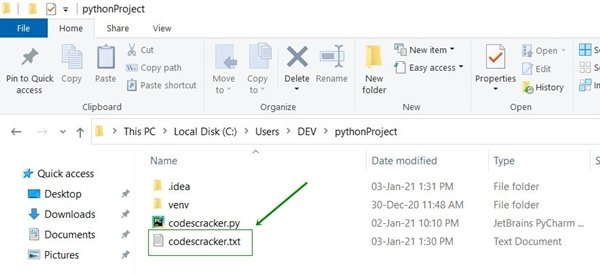And here is the snapshot of the opened file "codescracker.txt":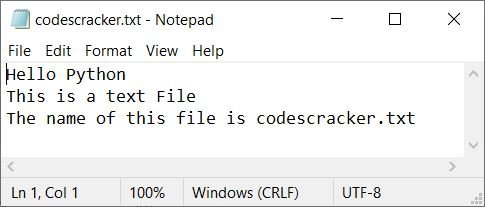Now let's create some Python programs to do the task of counting characters, vowels, spaces, etc. in this text file.

## Count Vowels in a Text File

The question is: write a Python program to count the number of vowels present in a file. The program given below is the answer to this question:

```print("Enter the Name of File: ")
fileName = str(input())
fileHandle = open(fileName, "r")
tot = 0
vowels = ['a', 'e', 'i', 'o', 'u', 'A', 'E', 'I', 'O', 'U']

if char in vowels:
tot = tot+1
fileHandle.close()

print("\nTotal Vowels are:")
print(tot)```

Here is its sample run:Now enter the name of the file, say "codescracker.txt" (a newly created file as of the beginning of this article), and press ENTER to count and print the total number of vowels present in the content of this file, as shown in the snapshot given below:#### Modified Version of the Previous Program

Let's modify the previous program. This program uses "end" to skip printing an automatic newline. The "try-except" block is used for exception handling.

```print(end="Enter the Name of File: ")
fileName = str(input())
try:
fileHandle = open(fileName, "r")
tot = 0
vowels = ['a', 'e', 'i', 'o', 'u', 'A', 'E', 'I', 'O', 'U']

if char in vowels:
tot = tot+1
fileHandle.close()

if tot>1:
print("\nThere are " + str(tot) + " Vowels available in the File")
elif tot==1:
print("\nThere is only 1 Vowel available in the File")
else:
print("\nThere is no any Vowel available in the File!")
except IOError:
print("\nError Occurred!")
print("Either File doesn't Exist or Permission is not Allowed!")```

Here is its sample run with the same user input, saying "codescracker.txt" as the file's name: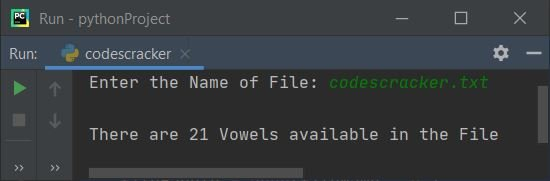Here is another sample run with user input, say "temp.txt" (a non-existing file):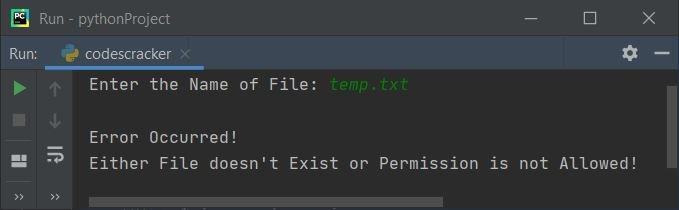## Count Consonants in a Text File

The question is: write a program in Python that counts the total number of consonants available in a text file. Here is its answer:

```print(end="Enter the Name of File: ")
fileName = str(input())
try:
fileHandle = open(fileName, "r")
tot = 0
vowels = ['a', 'e', 'i', 'o', 'u', 'A', 'E', 'I', 'O', 'U']

if char>='a' and char<='z':
if char not in vowels:
tot = tot+1
elif char>='A' and char<='Z':
if char not in vowels:
tot = tot+1

fileHandle.close()

if tot>1:
print("\nThere are " + str(tot) + " Consonants available in the File")
elif tot==1:
print("\nThere is only 1 Consonant available in the File")
else:
print("\nThere is no any Consonant available in the File!")
except IOError:
print("\nError Occurred!")```

Here is its sample run with the same file name, "codescracker.txt":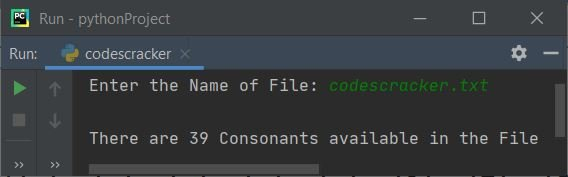This program is similar to the previous program. The only difference is in logical code; we've changed the following code:

```if char in vowels:
tot = tot+1```

with the block of code given below:

```if char>='a' and char<='z':
if char not in vowels:
tot = tot+1
elif char>='A' and char<='Z':
if char not in vowels:
tot = tot+1```

## Count New Lines in a Text File

To count the number of new lines in a text file, use the following Python program:

```print(end="Enter the Name of File: ")
fileName = str(input())
try:
fileHandle = open(fileName, "r")
tot = 0

if char=='\n':
tot = tot+1
fileHandle.close()

if tot>1:
print("\nThere are " + str(tot) + " New Lines available in the File")
elif tot==1:
print("\nThere is only 1 New Line available in the File")
else:
print("\nThere is no any New Line available in the File!")
except IOError:
print("\nError Occurred!")```

Here is its sample run with the same file name as created earlier: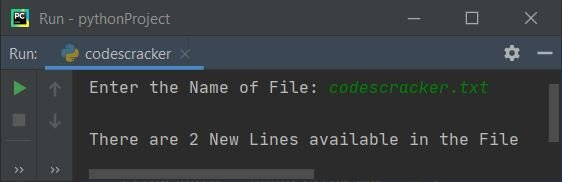The only difference between this program and the program given to count vowels in a text file is that we've changed the following block of code:

```if char in vowels:
tot = tot+1```

with the block of code given below:

```if char=='\n':
tot = tot+1```

## Counting Blank Spaces in a Text File

This program counts the total number of blank spaces available in a text file entered by the user at runtime.

```print(end="Enter the Name of File: ")
fileName = str(input())
try:
fileHandle = open(fileName, "r")
tot = 0

if char==' ':
tot = tot+1
fileHandle.close()

if tot>1:
print("\nThere are " + str(tot) + " Blank spaces available in the File")
elif tot==1:
print("\nThere is only 1 Blank space available in the File")
else:
print("\nThere is no any Blank space available in the File!")
except IOError:
print("\nError Occurred!")```

The snapshot given below shows the sample run of this program with the same file name, "codescracker.txt."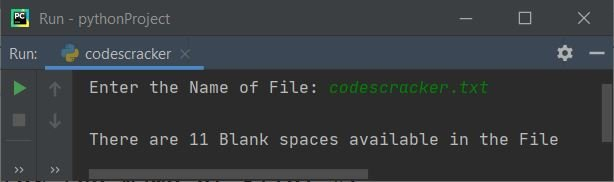## Count Total Characters in a Text File

This is the last program in this article. This program is created to count all the characters, or total number of characters, available in a text file.

```print(end="Enter the Name of File: ")
fileName = str(input())
try:
fileHandle = open(fileName, "r")
tot = 0

if char:
tot = tot+1
fileHandle.close()

if tot>1:
print("\nThere are " + str(tot) + " Characters available in the File")
elif tot==1:
print("\nThere is only 1 Character available in the File")
else:
print("\nThe File is empty!")
except IOError:
print("\nError Occurred!")```

Here is its sample run: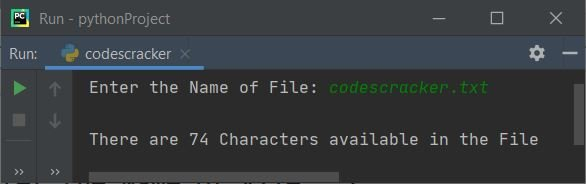Note: Out of "74" characters, there are "21" vowels, "39" consonants, "11" blank spaces, "2" new lines, and "1" dot (.)

Python Online Test

« Previous Program Next Program »